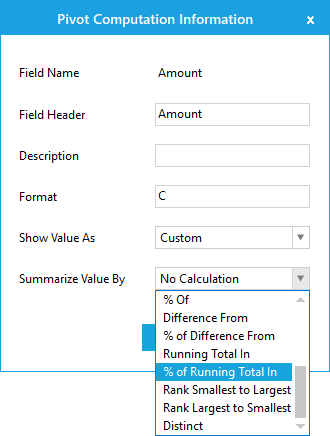# Summaries in Windows Forms Pivot Grid

24 Aug 20215 minutes to read

Summaries can be defined for the pivot calculation values in the pivot grid. Pivot grid control supports 19 built-in summary types to customize the summaries.

## Summary Types

Pivot grid summarizes the data for various data types by using the SummaryType enumerator. The SummaryType value should be defined while defining the PivotCalculation items using PivotComputationInfo class to specify the type of the summary. The following summary types can be set to a computation item.

Summary Type Description
DoubleTotalSum Computes the sum of double or integer values.
DoubleAverage Computes the simple average of double or integer values.
DoubleMaximum Computes the maximum of double or integer values.
DoubleMinimum Computes the minimum of double or integer values.
DoubleStandardDeviation Computes the standard deviation of double or integer values.
DoubleVariance Computes the variance of double or integer values.
Count Computes the count of double or integer values.
DecimalTotalSum Computes the sum of decimal values.
IntTotalSum Computes the sum of integer values.
Custom Specifies that you are using a custom SummaryBase object to define the calculation.

### Customizing summary type at run time

Summary type of pivot calculation values can be customized by using the pivot computation information dialog. While double clicking on a calculation item in the value layout section of pivot schema designer, the pivot computation information dialog will be displayed. The summary type of pivot calculation item can be changed by using the “Summarize Value By” combo box resides in the pivot computation information dialog as specified below.## Custom Summaries

PivotGrid allows to set custom summaries for pivot calculation values by creating a custom SummaryBase class. For creating a custom summary, a new class need to be added by inheriting the abstract class `SummaryBase`. Summary logics can be overridden by overriding the following methods: `Combine()`, `CombineSummary()`, `GetResult()`, `GetInstance()` and `Reset()`.

Refer to the below code sample to define custom summaries for pivot calculation values.

``````public partial class Form1 : Form
{
public Form1()
{
......
pivotGridControl1.PivotCalculations.Add(new PivotComputationInfo { FieldName = "Amount", Format = "C", SummaryType = SummaryType.Custom, Summary = new CustomSummaryBase() });
......
}
}

public class CustomSummaryBase : SummaryBase
{
private double mTotalValue;

public override void Combine(object other)
{
mTotalValue += (double)other;
}

public override void CombineSummary(SummaryBase other)
{
CustomSummaryBase customSummaryBase = other as CustomSummaryBase;

if (customSummaryBase != null)
{
mTotalValue += customSummaryBase.mTotalValue;
}
}

public override SummaryBase GetInstance()
{
return new CustomSummaryBase();
}

public override object GetResult()
{
return mTotalValue / 3.33333;
}

public override void Reset()
{
mTotalValue = 0;
}
}``````
``````Partial Public Class Form1
Inherits Form
Public Sub New()
......
pivotGridControl1.PivotCalculations.Add(New PivotComputationInfo With {.FieldName = "Amount", .Format = "C", .SummaryType = SummaryType.Custom, .Summary = New CustomSummaryBase()})
......
End Sub
End Class

Public Class CustomSummaryBase
Inherits SummaryBase
Private mTotalValue As Double

Public Overrides Sub Combine(ByVal other As Object)
mTotalValue += CDbl(other)
End Sub

Public Overrides Sub CombineSummary(ByVal other As SummaryBase)
Dim customSummaryBase As CustomSummaryBase = TryCast(other, CustomSummaryBase)

If customSummaryBase IsNot Nothing Then
mTotalValue += customSummaryBase.mTotalValue
End If
End Sub

Public Overrides Function GetInstance() As SummaryBase
Return New CustomSummaryBase()
End Function

Public Overrides Function GetResult() As Object
Return mTotalValue / 3.33333
End Function

Public Overrides Sub Reset()
mTotalValue = 0
End Sub
End Class``````